# 利用 Scikit-Learn 和 Spark 预测 Airbnb 的 listing 价格

## 开始

• listings.csv.gz：详细的 listing 数据，包含每个 listing 的各种属性，比如，卧室数目、浴室数目、位置等；
• calendar.csv.gz：每个 listing 的日历信息；
• reviews.csv.gz ：listing 的浏览数据；
• neighborhoods and GeoJSON files：同城邻居的地图和详细信息。

## 扫描数据和清洗数据

%matplotlib inline
import pandas as pd
import numpy as np
from sklearn import ensemble
from sklearn import linear_model
from sklearn.grid_search import GridSearchCV
from sklearn import preprocessing
from sklearn.cross_validation import train_test_split
import sklearn.metrics as metrics
import matplotlib.pyplot as plt
from collections import Counter

LISTINGSFILE = '/mapr/tmclust1/user/mapr/pyspark-learn/airbnb/listings.csv'

cols = ['price',
'accommodates',
'bedrooms',
'beds',
'neighbourhood_cleansed',
'room_type',
'cancellation_policy',
'instant_bookable',
'reviews_per_month',
'number_of_reviews',
'availability_30',
'review_scores_rating'
]

# read the file into a dataframe
df = pd.read_csv(LISTINGSFILE, usecols=cols)

neighborhood_cleansed 列是房主的邻居信息。你会看到这些信息分布不均衡，通过如下的图看出分布是个曲线，末尾的数量高，而靠左边非常少。总体来说，房主的邻居信息分布合理。

nb_counts = Counter(df.neighbourhood_cleansed)
tdf = pd.DataFrame.from_dict(nb_counts, orient='index').sort_values(by=0)
tdf.plot(kind='bar')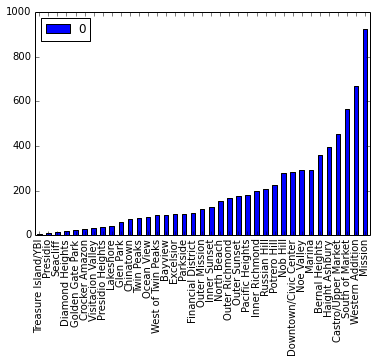number_reviews'和 reviews_per_month 两列看起来要去掉大量的 NaN 值（Python 中 NaN 值就是 NULL）。我们把 reviews_per_month 为 NaN 值的地方设置为 0，因为在某些数据分析中这些数据是有意义的。

# first fixup 'reviews_per_month' where there are no reviews
df['reviews_per_month'].fillna(0, inplace=True)

# just drop rows with bad/weird values
# (we could do more here)
df = df[df.bedrooms != 0]
df = df[df.beds != 0]
df = df[df.price != 0]
df = df.dropna(axis=0)

df = df[df.bedrooms == 1]

# remove the $from the price and convert to float df['price'] = df['price'].replace('[\$,)]','',  \
regex=True).replace('[(]','-', regex=True).astype(float)

## 类别变量处理

neighborhood_cleansed 列是邻居的名字，string 类型。scikit-learn 中的回归分析只接受数值类型的列。对于这类变量，使用 Pandas 的 get_dummies 转换成虚拟变量，这个处理过程也叫“one hot”编码，每个 listing 行都包含一个“1”对应她／他的邻居。我们用类似的方法处理 cancellation_policy 和 room_type 列。

instant_bookable 列是个 boolean 类型的值。
# get feature encoding for categorical variables
n_dummies = pd.get_dummies(df.neighbourhood_cleansed)
rt_dummies = pd.get_dummies(df.room_type)
xcl_dummies = pd.get_dummies(df.cancellation_policy)

# convert boolean column to a single boolean value indicating whether this listing has instant booking available
ib_dummies = pd.get_dummies(df.instant_bookable, prefix="instant")
ib_dummies = ib_dummies.drop('instant_f', axis=1)

# replace the old columns with our new one-hot encoded ones
alldata = pd.concat((df.drop(['neighbourhood_cleansed', \
'room_type', 'cancellation_policy', 'instant_bookable'], axis=1), \
n_dummies.astype(int), rt_dummies.astype(int), \
xcl_dummies.astype(int), ib_dummies.astype(int)), \
axis=1)
allcols = alldata.columns

scattercols = ['price','accommodates', 'number_of_reviews', 'reviews_per_month', 'beds', 'availability_30', 'review_scores_rating']
axs = pd.scatter_matrix(alldata[scattercols],
figsize=(12, 12), c='red')

(点击放大图像)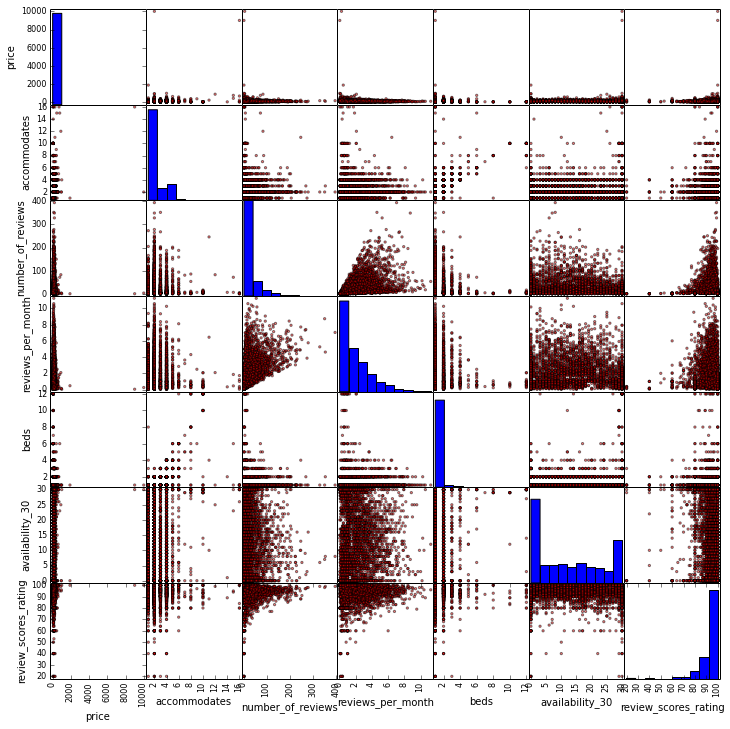scatter_matrix 的输出结果发现并没有什么明显的问题。最相近的特征应该是 beds 和 accommodates。

## 开始预测

scikit-learn 最大的优势是我们可以在相同的数据集上做不同的线性模型，这可以给我们一些调参的提示。我们开始使用其中的六种：vanilla linear regression, ridge and lasso regressions, ElasticNet, bayesian ridge 和 Orthogonal Matching Pursuit。

rs = 1
ests = [ linear_model.LinearRegression(), linear_model.Ridge(),
linear_model.Lasso(), linear_model.ElasticNet(),
linear_model.BayesianRidge(), linear_model.OrthogonalMatchingPursuit() ]
ests_labels = np.array(['Linear', 'Ridge', 'Lasso', 'ElasticNet', 'BayesRidge', 'OMP'])
errvals = np.array([])

X_train, X_test, y_train, y_test = train_test_split(alldata.drop(['price'], axis=1),
alldata.price, test_size=0.2, random_state=20)

for e in ests:
e.fit(X_train, y_train)
this_err = metrics.median_absolute_error(y_test, e.predict(X_test))
#print "got error %0.2f" % this_err
errvals = np.append(errvals, this_err)

pos = np.arange(errvals.shape)
srt = np.argsort(errvals)
plt.figure(figsize=(7,5))
plt.bar(pos, errvals[srt], align='center')
plt.xticks(pos, ests_labels[srt])
plt.xlabel('Estimator')
plt.ylabel('Median Absolute Error')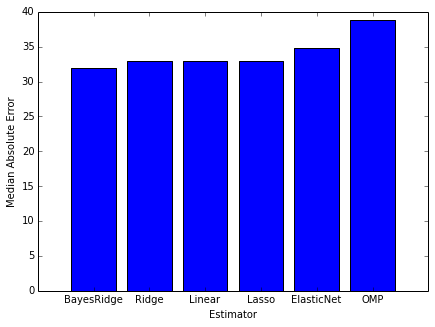n_est = 300

tuned_parameters = {
"n_estimators": [ n_est ],
"max_depth" : [ 4 ],
"learning_rate": [ 0.01 ],
"min_samples_split" : [ 1 ],
"loss" : [ 'ls', 'lad' ]
}

gbr = ensemble.GradientBoostingRegressor()
clf = GridSearchCV(gbr, cv=3, param_grid=tuned_parameters,
scoring='median_absolute_error')
preds = clf.fit(X_train, y_train)
best = clf.best_estimator_

# plot error for each round of boosting
test_score = np.zeros(n_est, dtype=np.float64)

train_score = best.train_score_
for i, y_pred in enumerate(best.staged_predict(X_test)):
test_score[i] = best.loss_(y_test, y_pred)

plt.figure(figsize=(12, 6))
plt.subplot(1, 2, 1)
plt.plot(np.arange(n_est), train_score, 'darkblue', label='Training Set Error')
plt.plot(np.arange(n_est), test_score, 'red', label='Test Set Error')
plt.legend(loc='upper right')
plt.xlabel('Boosting Iterations')
plt.ylabel('Least Absolute Deviation')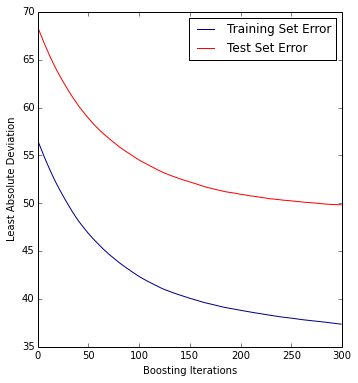from pyspark import SparkContext, SparkConf
from spark_sklearn import GridSearchCV

conf = SparkConf()
sc = SparkContext(conf=conf)
clf = GridSearchCV(sc, gbr, cv=3, param_grid=tuned_parameters, scoring='median_absolute_error')

Machine: HP DL380 G6

Memory: 128G

CPU: (2x) Intel X5560

Disk: (6x) 1TB 7200RPM disks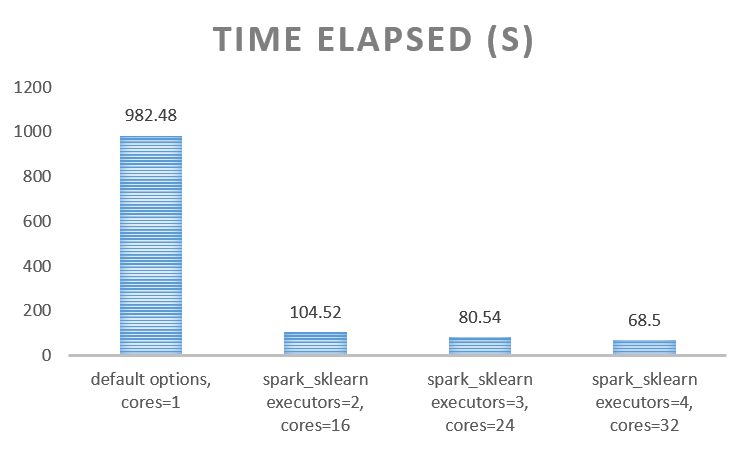feature_importance = clf.best_estimator_.feature_importances_
feature_importance = 100.0 * (feature_importance / feature_importance.max())
sorted_idx = np.argsort(feature_importance)
pos = np.arange(sorted_idx.shape) + .5
pvals = feature_importance[sorted_idx]
pcols = X_train.columns[sorted_idx]
plt.figure(figsize=(8,12))
plt.barh(pos, pvals, align='center')
plt.yticks(pos, pcols)
plt.xlabel('Relative Importance')
plt.title('Variable Importance')

(点击放大图像)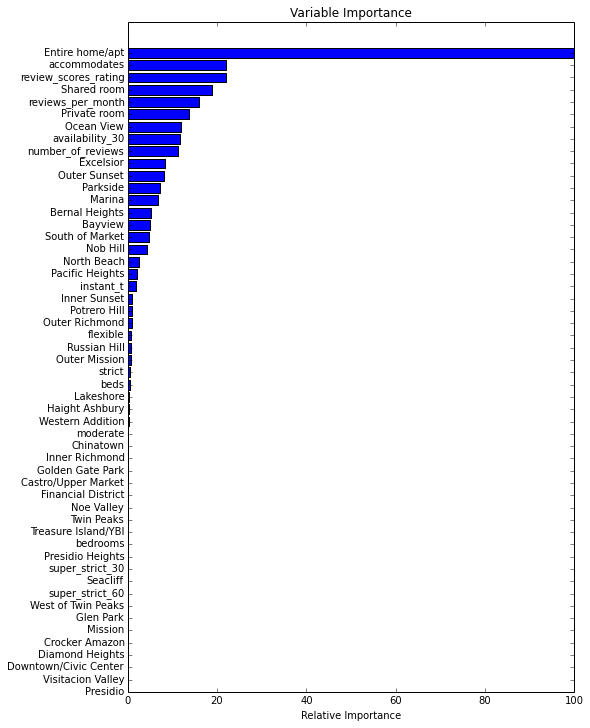## 结论

• GradientBoostingRegressor 等集成方法比单个方法得出的结果要好；
• 使用 GridSearchCV 函数可以测试更多的超参数组合来得到更优的结果；
• 使用 spark-sklearn 能更好节约 CPU 和时间，减少评估错误。

## 译者介绍